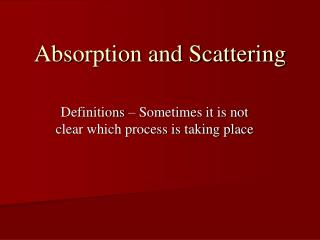DownloadDownload PresentationAbsorption and Scattering

# Absorption and Scattering

Download Presentation## Absorption and Scattering

- - - - - - - - - - - - - - - - - - - - - - - - - - - E N D - - - - - - - - - - - - - - - - - - - - - - - - - - -
##### Presentation Transcript

1. Absorption and Scattering Definitions – Sometimes it is not clear which process is taking place

2. Absorption and Scattering • Scattering: Photon interacts with the scatterer and emerges in a new direction with (perhaps) a slightly different frequency • No destruction of the photon in the sense that energy is added to the kinetic pool • Absorption: Photon interacts and is destroyed and its energy is converted (at least partially) into kinetic energy of the particles composing the gas. • Scattering is mostly independent of the thermal properties of the gas and depends mostly on the radiation field. Absorption and Scattering

3. Absorption and Scattering • Absorption processes depend on the thermodynamic properties of the gas as they feed energy directly into the gas. • NB: thermal emission couples the thermodynamic quantities directly to the radiation field! Absorption and Scattering

4. Scattering Processes • Bound-Bound Transitions followed by a direct reverse transition: • a →b : b → a • There can be a small E as the energy states have finite width (substates) • E ~0 and “only” the direction changed! • Photon scattering by a free electron (Thomson) or molecule (Rayleigh) • Thomson: 0.6655 (10-24) Ne • Rayleigh: λ-4 Absorption and Scattering

5. Absorption Processes • Photoionization / bound-free transition • Excess energy goes into KE • Inverse Process: Radiative Recombination • Free-Free Absorption: an electron moving in the field of an ion absorbs a photon causing a shift in the “orbit” (hyperbolic). • Inverse Process: Bremsstrahlung • Bound-Bound Photoexcitation followed immediately by a collisional de-excitation ==> photon energy shared by partners and goes into the kinetic pool. • Inverse Process: ----------- • Photo-excitation followed immediately by a collisional ionization. • Inverse Process: Collisional Recombination Absorption and Scattering

6. Caveats • These lists are not exhaustive. In many cases the line between the two processes is not clear, especially with bound-free events. Absorption and Scattering

7. Absorption Coefficient • Absorption Coefficient κν per gram of stellar material such that: • A differential element of material of cross section dA and length ds absorbs an amount of energy from a beam of specific intensity Iν (incident normal to the ends of the element): • dEν = ρ κν Iν dω dt dA ds dν Absorption and Scattering

8. Scattering Coefficient • Similarly, σν governs the amount of energy scattered out of the beam. dEν = ρ σν Iν dω dt dA ds dν • We assume that both κν and σν have no azimuthal dependence. The combined effect of κν and σν is to remove energy from the beam. • The total extinction coefficient is: Κν = κν + σν • Κν is called the “mass absorption coefficient” Absorption and Scattering

9. The Simple Slab dIν = -ρκνIνdz Iν: ergs Hz-1 s-1 cm-1 sterad-1 κν: cm2 gm-1 ρκν : cm-1 Iν +dIν (dIν < 0) Z+dZ (dZ > 0) Z Iν Absorption and Scattering

10. The Simple Slab Continued • In the above 1 and 2 are arbitrary limits • Let us now integrate over the slab (assign physically meaningful values to the integration limits). We want the “output” at the top (Z=0) coming from depth X in the slab (star?!) • Iν1 = Initial intensity = Iν(X) • Iν2 = Output Intensity • Z1 = Level of Origin • Z2 = Level of Output (= 0) Absorption and Scattering

11. The Optical Depth The Dimensionless Quantity τ Note that τ and X increase in opposite directions. Boundary Conditions: At the surface τν1 ≡ 0 and at the “base” X1 ≡ 0. Therefore: Absorption and Scattering

12. Optical Depth Surfaces and Probability • The optical depth of a slab determines how much light escapes from a given level: • If τ = 2 then I = I0e-2 ==> 0.135 of I0 escapes • The usual definition of “continuum” (AKA the “surface” of a star) is where a photon has a 50% chance of escaping: • e-τ = 0.5 ==> τ = 0.693 • An exercise in atmospheric extinction. A cloud can easily contribute 3 magnitudes of extinction: • 1/(2.512)3 = e-τ ==> τ = 2.763. Absorption and Scattering

13. Emission Processes If there is a sink then there must be a source! • Emission coefficient: jν • dEν = ρ jν dω dν dt dA ds • Or per unit mass in an incremental volume: • dEν = jν dω dν dt • Let us consider thermal emission. Consider a cavity of uniform temperature T which is a blackbody. This demands • jνt= κνBν(T) – Kirchoff-Planck Law • κν is absorption only • Strict Thermodynamic Equilibrium applies • Thermal absorption and emission independent of angle. Absorption and Scattering

14. TE and Stellar Atmospheres • Energy is transported in a stellar atmosphere • This means the radiation field is anisotropic • There is also a temperature gradient which is demanded by the energy flow (2nd Law) • Strict Thermodynamic Equilibrium cannot hold! • For convenience we shall assume that local thermodynamic equilibrium holds ==> T, Ne, etc locally determine occupation numbers. Absorption and Scattering